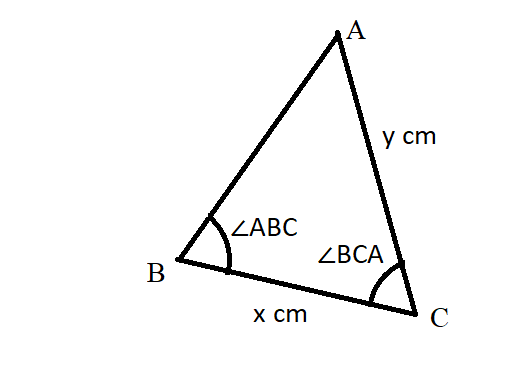# PQRS is a parallelogram. m∠Q = 11x + 13 and m∠S = 15x - 23. Find the measure of ∠P.Question
Non-right triangles and trigonometryPQRS is a parallelogram. $$\displaystyle{m}∠{Q}={11}{x}+{13}$$ and $$\displaystyle{m}∠{S}={15}{x}-{23}$$. Find the measure of $$\displaystyle∠{P}$$.2021-01-09
A parallelogram has opposite angles that are congruent so:
$$\displaystyle∠{Q}≅∠{S}$$
$$\displaystyle{m}∠{Q}={m}∠{S}$$
11x+13=15x−23
11x=15x−36
−4x=−36
x=9
So, $$\displaystyle{m}∠{Q}={11}{\left({9}\right)}+{13}={112}°.$$
A parallelogram has consecutive angles that are supplementary:
$$\displaystyle{m}∠{P}+{m}∠{Q}={180}°$$
$$\displaystyle{m}∠{P}+{112}°={180}°$$
$$\displaystyle{m}∠{P}={68}°$$

### Relevant QuestionsGiven:
A parallelogram with diagonals equal to 56cm and 34cm. The intersect of the angles is $$\displaystyle{120}^{\circ}$$. Find the shorter side's length.Find the shorter diagonal of a parallelogram, if
l=18cm
w=26cm
$$\displaystyle\angle{a}={39}^{\circ}$$The base of a pyramid covers an area of 13.0 acres (1 acre =43,560 $$\displaystyle{f}{t}^{{2}}$$) and has a height of 481 ft . Ifthe volume of a pyramid is given by the expression V =(1/3)bh, where b is the area of the base and his the height, find the volume of this pyramid in cubicmeters.In triangle ABC, angle B is five times as large as angle A. The measure of angle C is 2° less than that of angle A. Find the measures of the angles. (Hint: The sum of the angle measures is 180°.)In $$\displaystyle△{P}{Q}{R},{m}∠{P}={46}{x}$$ and $$\displaystyle{m}∠{R}={7}{x}$$,
a. Write and solve an equation to find the measure of each angle.
b. Classify the triangle based on its angles.[Triangle] Determine the measure of each interior angle of △MAN and classify the triangle. m m /trnglMAN is a(n)_______________ triangle.Suppose that $$\displaystyle{m}\angle{B}{C}{A}={70}^{\circ}$$, and x=33 cm and y = 47 cm. What is the degree measure of $$\displaystyle\angle{A}{B}{C}$$?A triangle is given with one side equal to 18m (a) and angles laying on this side equal to $$\displaystyle{48}^{\circ}$$ (A) and $$\displaystyle{37}^{\circ}$$ (B). Find the ramaining angle (C) and sides (b and c).